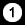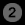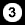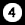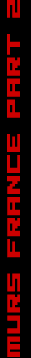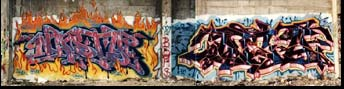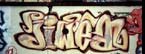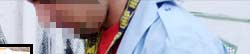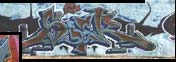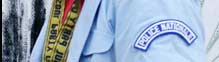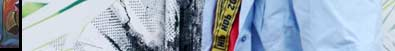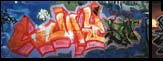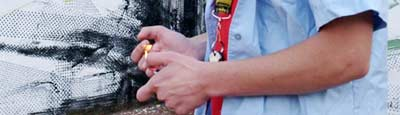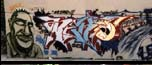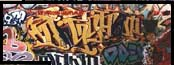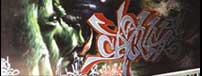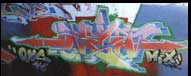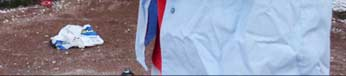N A S T Y   D E A   /   J I W E A   S D K   /   C A N 2   S W E T   /   S E B   F M K   /   S O E R   C V  J A Y O N E   /   L E G Z   P 1 9   /   D E K O   /   J A B A   J N C   /   A C U Z   1 3 2   /   N E FA Z E   V M D